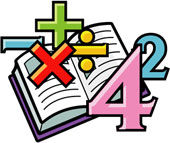#### Big Idea (Grade 1)

This video illustrates ways to the model or think about the operations of addition and subtraction. Included are combining groups, the take-away model, the number line model, family of facts and the missing addend.#### Learning the Basic Facts of Addition (Grade 1)

This video suggests strategies for learning the basic addition facts. Included are references to the number line, ten-frame, fact families and doubles.#### Learning the Basic Facts of Subtraction (Grade 1)

This video suggests strategies for learning the basic facts of subtraction including counting back,number bonds and sissing addends.#### Place Value  (Grade 2)

Base ten blocks and place value disks are used to help students visualize and understand place value.#### Adding and Subtracting - No Regrouping (Grade 2)

This video gives examples of how to add and subtract multidigt numbers that do not require regrouping. Place value disks and base ten materials are used to explain the process.This video explains the process of adding multi-digit numbers. Place value disks are used to explain the process. Examples are given.#### Subtraction Using Regrouping  (Grade 2/3)

The video gives examples of using the standard algorithm for multi-digit subtraction. Includes a short discussion of why the algorithm works using place value disks.#### Big Idea (Grade 2)

Video talks about the difference models for multiplication and division including the array model, division as missing multiplier and fact families.#### Learning the Basic Facts of Multiplication and Division (Grade 2)

Video covers skip counting, singing the facts, multiplying by 9, commutative property of multiplication and fact families.#### Keeping it Simple (Grade 3)

First of two videos on mult-digit multiplication. Place value disks and partial products are used to lead into the standard algorithm for multiplication.#### Multi-digit Multiplication - Part 2